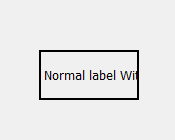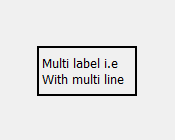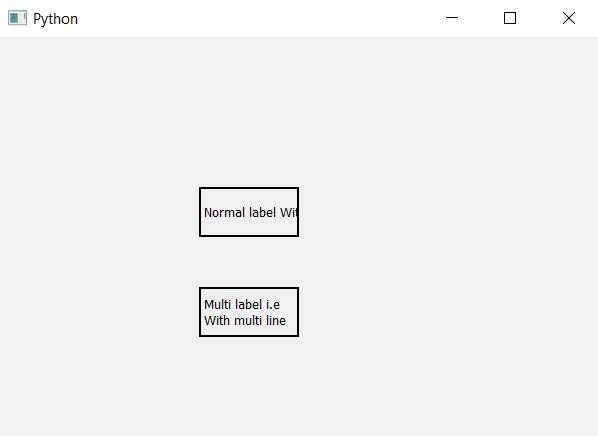Related Articles
PyQt5 – Multi line label
• Last Updated : 06 May, 2020

In this article we will see how we can create a multi-line label, when we create a label and set text to it and if the text length is greater then the length of the label extra text did not show, multi-line label make that extra text go into the other line. Below is the representation of how normal and multi-line label looks likeIn order to do this we will use `setWordWrap` method.

Syntax : label.setWordWrap(True)

Argument : It takes bool as argument

Return : None

Below is the implementation

 `# importing libraries``from` `PyQt5.QtWidgets ``import` `*` `from` `PyQt5 ``import` `QtCore, QtGui``from` `PyQt5.QtGui ``import` `*` `from` `PyQt5.QtCore ``import` `*` `import` `sys`` ` ` ` `class` `Window(QMainWindow):`` ` `    ``def` `__init__(``self``):``        ``super``().__init__()`` ` `        ``# setting title``        ``self``.setWindowTitle(``"Python "``)`` ` `        ``# setting geometry``        ``self``.setGeometry(``100``, ``100``, ``600``, ``400``)`` ` `        ``# calling method``        ``self``.UiComponents()`` ` `        ``# showing all the widgets``        ``self``.show()`` ` `    ``# method for widgets``    ``def` `UiComponents(``self``):`` ` `        ``# creating label``        ``label1 ``=` `QLabel(``"Normal label With out multi line"``, ``self``)`` ` `        ``# setting geometry to the label``        ``label1.setGeometry(``200``, ``150``, ``100``, ``50``)`` ` `        ``# adding border to the label``        ``label1.setStyleSheet(``"border : 2px solid black;"``)`` ` `        ``# creating label``        ``label2 ``=` `QLabel(``"Multi label i.e With multi line"``, ``self``)`` ` `        ``# setting geometry to the label``        ``label2.setGeometry(``200``, ``250``, ``100``, ``50``)`` ` `        ``# adding border to the label``        ``label2.setStyleSheet(``"border : 2px solid black;"``)`` ` `        ``# making it multi line``        ``label2.setWordWrap(``True``)`` ` ` ` `# create pyqt5 app``App ``=` `QApplication(sys.argv)`` ` `# create the instance of our Window``window ``=` `Window()`` ` `window.show()`` ` `# start the app``sys.exit(App.``exec``())`

Output :Attention geek! Strengthen your foundations with the Python Programming Foundation Course and learn the basics.

To begin with, your interview preparations Enhance your Data Structures concepts with the Python DS Course.

My Personal Notes arrow_drop_up